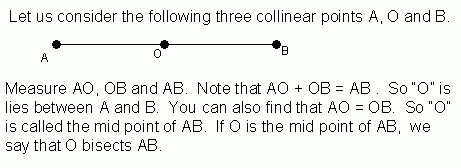Email us to get an instant 20% discount on highly effective K-12 Math & English kwizNET Programs!

#### Online Quiz (WorksheetABCD)

Questions Per Quiz = 2 4 6 8 10

### MEAP Preparation - Grade 7 Mathematics4.31 Midpoint of Line Segment

 Midpoint of a line segment is the point on the segment that divides the segment into two congruent segments. If B is the midpoint of line AC, then AB = BC. A segment bisector is a point, ray, line, line segment or plane that intersects the segment at its midpoint. A midpoint or a segment bisector bisects a segment. ABDirections: Answer the following questions. Also write at least five examples of your own.
 Q 1: What is the mid point of EF whose length is 9 cm?9 cm13 cm4.5 cm Q 2: What is the mid point of GH whose length is 11 cm?7.5 cm15 cm5.5 cm Q 3: AO = OB, if AO = 2.5 cm, then OB = ?0 cm5 cm2.5 cm Q 4: AO = OB, if OB =10 cm, then AO = ?10 cm0 cm20 cm Question 5: This question is available to subscribers only! Question 6: This question is available to subscribers only!